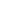Share

# Hertz

Hertz (abbreviated: Hz) is the standard unit of measurement used for measuring frequency. Since frequency is measured in cycles per second, one hertz equals one cycle per second.

Hertz is used commonly used to measure wave frequencies, such as sound waves, light waves, and radio waves. For example, the average human ear can detect sound waves between 20 and 20,000 Hz. Sound waves close to 20 Hz have a low pitch and are called "bass" frequencies. Sound waves above 5,000 Hz have a high pitch and are called "treble" frequencies.

While hertz can be used to measure wave frequencies, it is also used to measure the speed of computer processors. For example, each CPU is rated at a specific clock speed. This number indicates how many instruction cycles the processor can perform each second. Since modern processors can perform millions or even billions of instructions per second, clock speeds are typically measured in megahertz or gigahertz.

Abbeviation: Hz

Published: 2009

Definition from the PC Glossary
https://pc.net/glossary/hertz